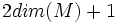# Stably parallelizable iff framable in large codimension

Suppose$M$ is a differential manifold. Then, the following are equivalent:
•$M$ is a stably parallelizable manifold i.e. its tangent bundle is a stably trivial vector bundle
• There exists a manifold$N$ such that$M$ can be embedded as a framable submanifold of$N$
• There exists a manifold$N$ of dimension at most$2dim(M) + 1$, such that$M$ can be embedded as a framable submanifold of$N$